# Social network-analysis-in-python

17. Mar 2014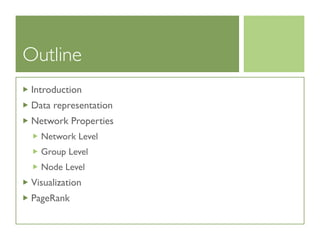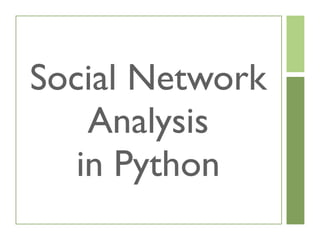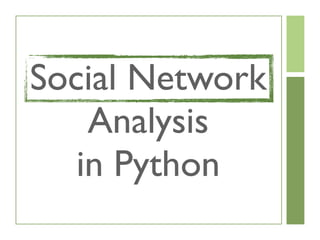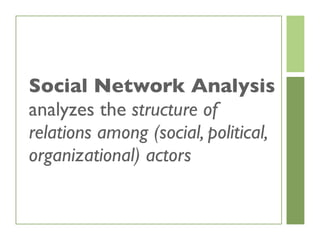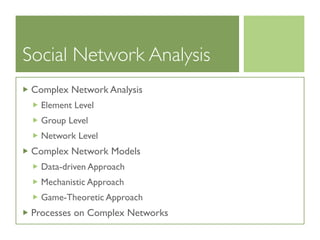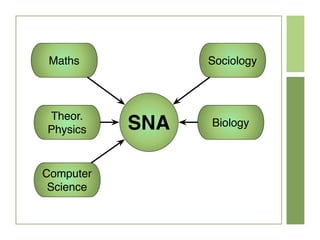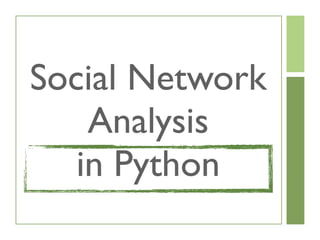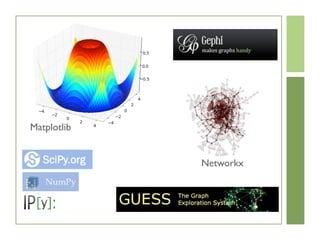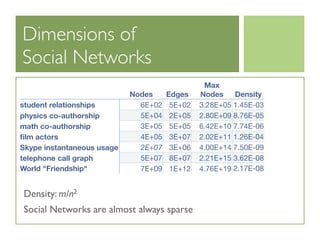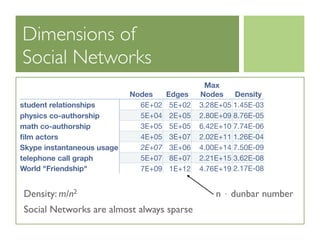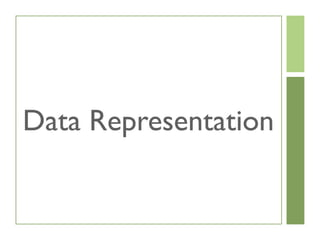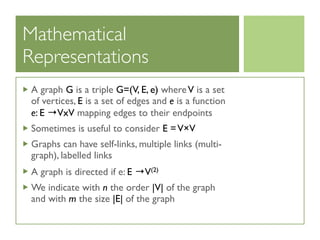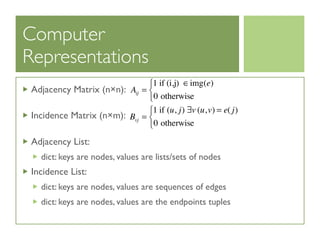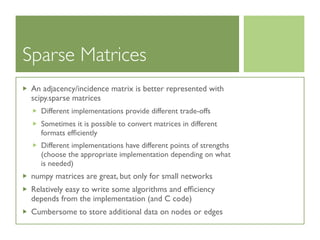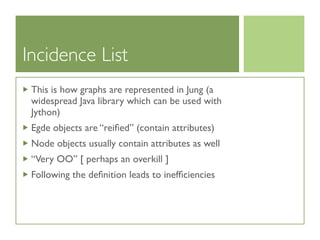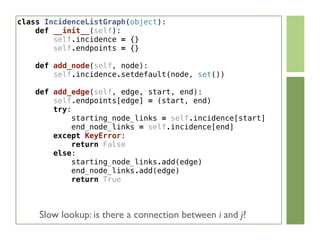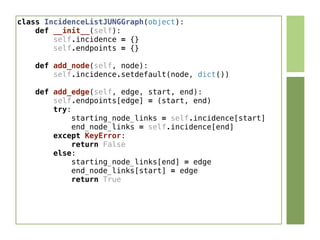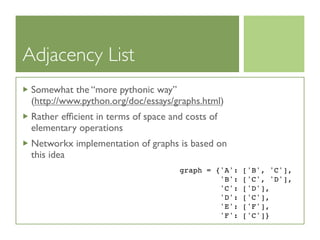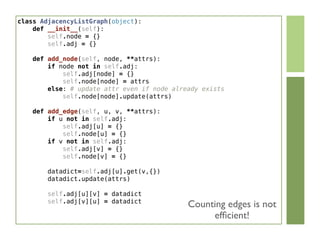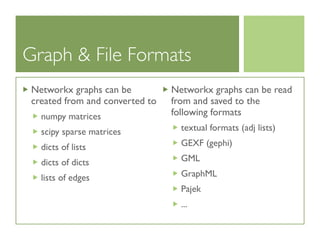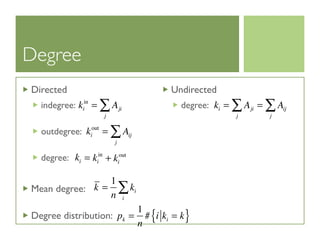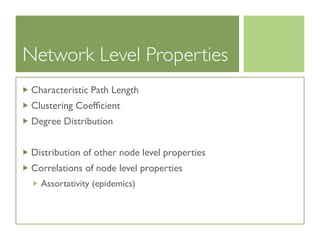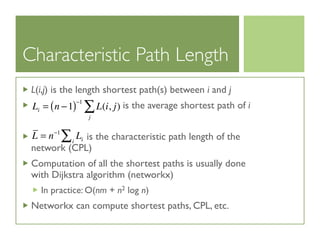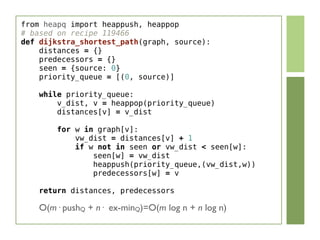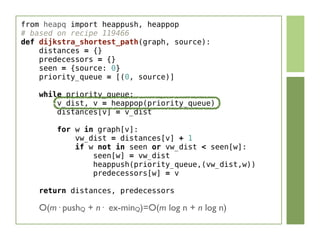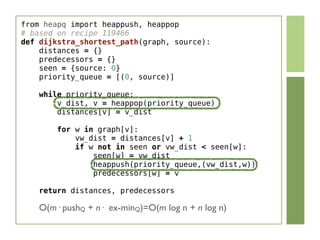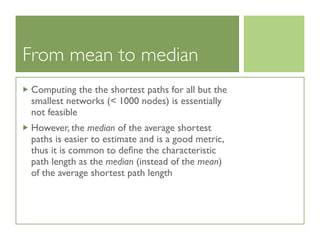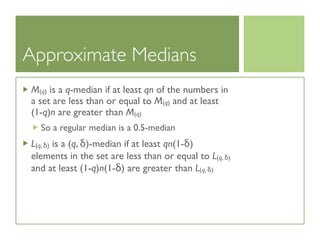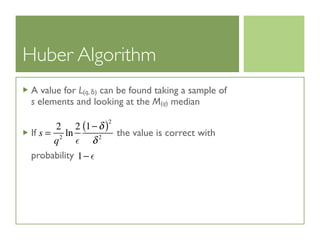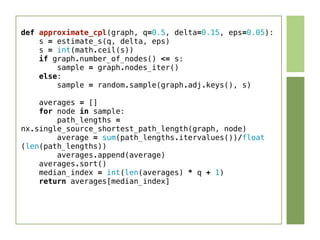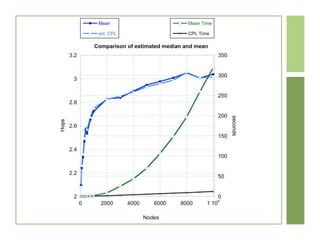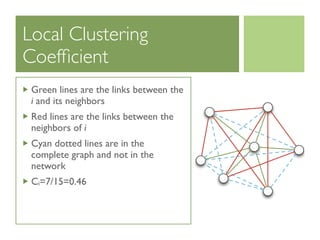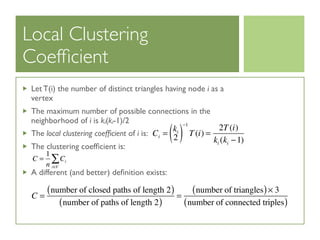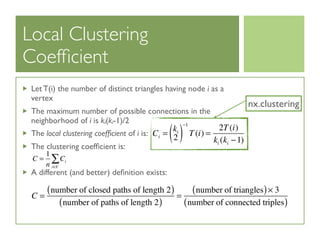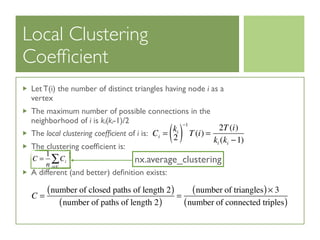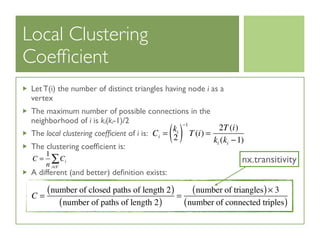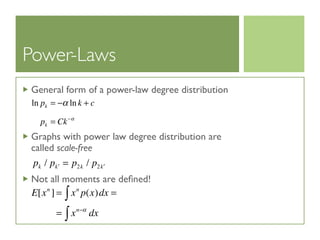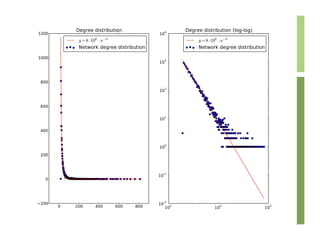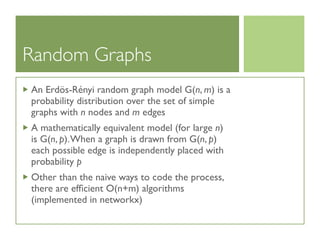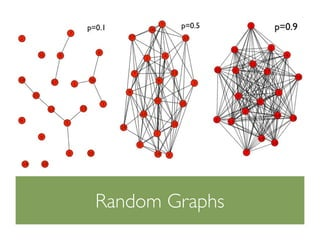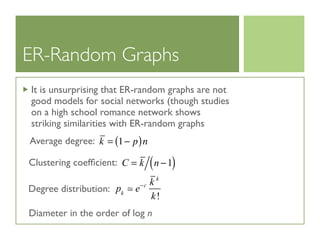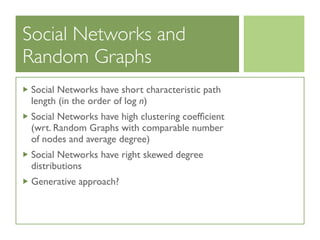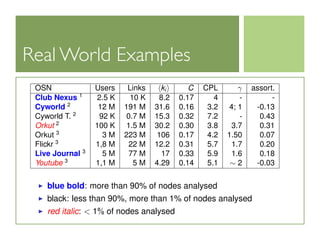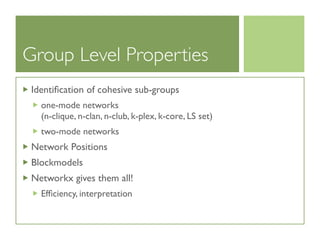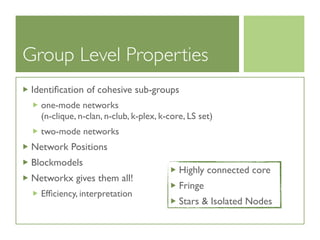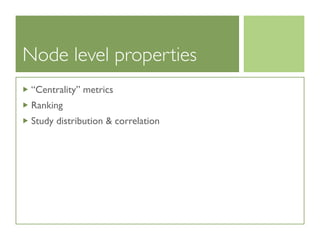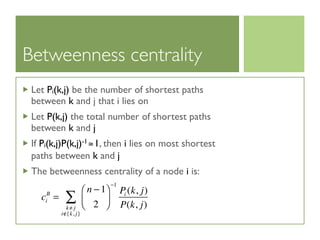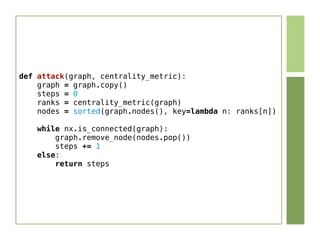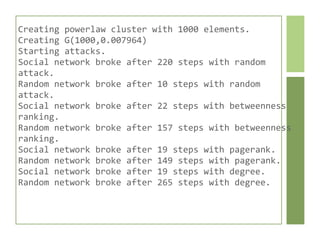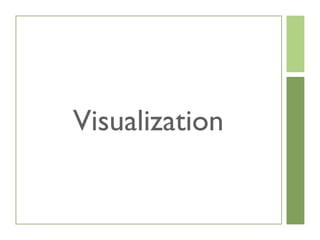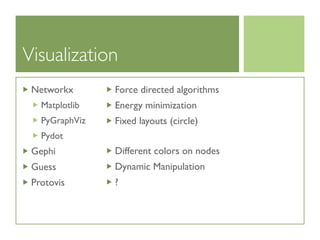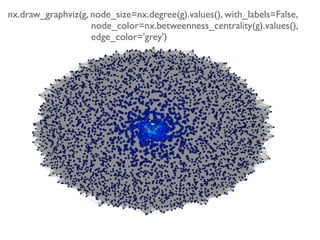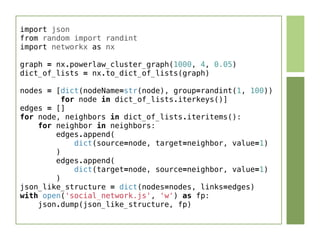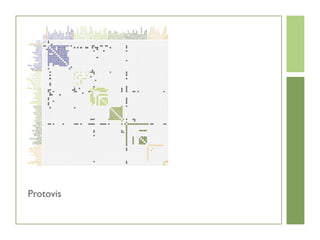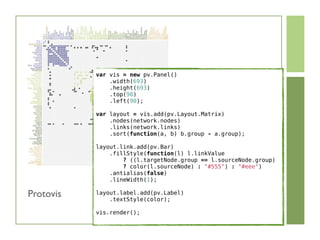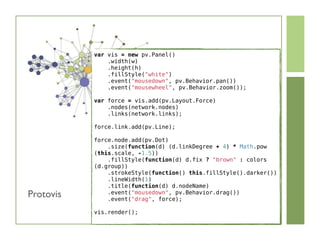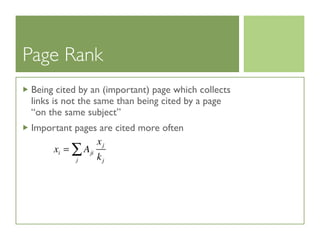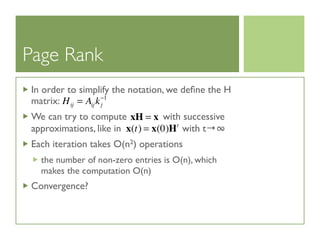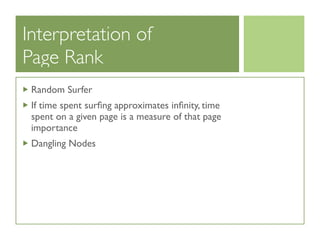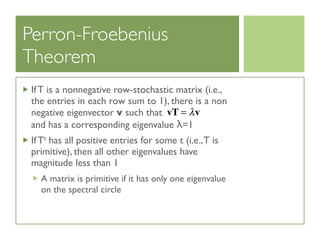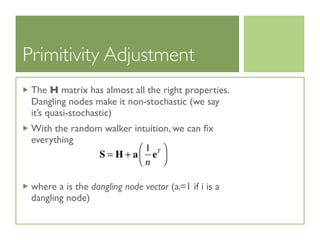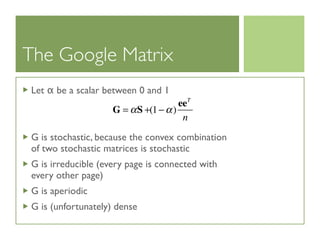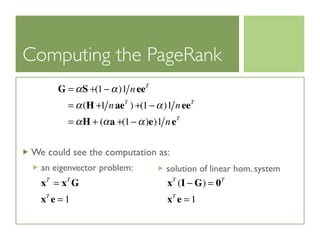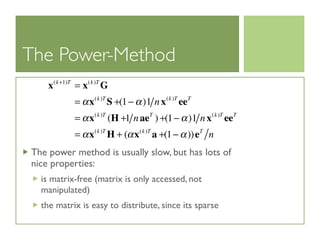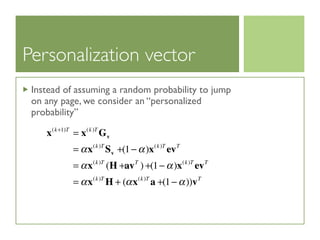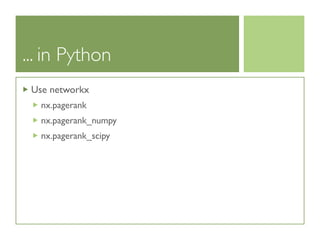1 von 80

### Social network-analysis-in-python

• 1. Social Network Analysis in Python Enrico Franchi (efranchi@ce.unipr.it) Dipartimento Ingegneria dell’Informazione Università degli Studi di Parma
• 2. Outline Introduction Data representation Network Properties Network Level Group Level Node Level Visualization PageRank
• 5. “A social network is a ﬁnite set of actors and the relations deﬁned on them”
• 6. “A social network is a ﬁnite set of actors and the relations deﬁned on them” NETWORK
• 7. “A social network is a ﬁnite set of actors and the relations deﬁned on them” NETWORK PEOPLE [ACTORS]
• 8. “A social network is a ﬁnite set of actors and the relations deﬁned on them” NETWORK PEOPLE [ACTORS] CONNECTIONS [RELATIONS]
• 11. Social Network Analysis analyzes the structure of relations among (social, political, organizational) actors
• 12. Social Network Analysis Complex Network Analysis Element Level Group Level Network Level Complex Network Models Data-driven Approach Mechanistic Approach Game-Theoretic Approach Processes on Complex Networks
• 17. Dimensions of Social Networks Nodes Edges Max Nodes Density student relationships physics co-authorship math co-authorship ﬁlm actors Skype instantaneous usage telephone call graph World "Friendship" 6E+02 5E+02 3.28E+05 1.45E-03 5E+04 2E+05 2.80E+09 8.76E-05 3E+05 5E+05 6.42E+10 7.74E-06 4E+05 3E+07 2.02E+11 1.26E-04 2E+07 3E+06 4.00E+14 7.50E-09 5E+07 8E+07 2.21E+15 3.62E-08 7E+09 1E+12 4.76E+19 2.17E-08 Density: m/n2 Social Networks are almost always sparse
• 18. Dimensions of Social Networks Nodes Edges Max Nodes Density student relationships physics co-authorship math co-authorship ﬁlm actors Skype instantaneous usage telephone call graph World "Friendship" 6E+02 5E+02 3.28E+05 1.45E-03 5E+04 2E+05 2.80E+09 8.76E-05 3E+05 5E+05 6.42E+10 7.74E-06 4E+05 3E+07 2.02E+11 1.26E-04 2E+07 3E+06 4.00E+14 7.50E-09 5E+07 8E+07 2.21E+15 3.62E-08 7E+09 1E+12 4.76E+19 2.17E-08 Density: m/n2 Social Networks are almost always sparse n ⋅ dunbar number
• 20. Mathematical Representations A graph G is a triple G=(V, E, e) whereV is a set of vertices, E is a set of edges and e is a function e: E →VxV mapping edges to their endpoints Sometimes is useful to consider E =V×V Graphs can have self-links, multiple links (multi- graph), labelled links A graph is directed if e: E →V(2) We indicate with n the order |V| of the graph and with m the size |E| of the graph
• 21. Computer Representations Adjacency Matrix (n×n): Incidence Matrix (n×m): Adjacency List: dict: keys are nodes, values are lists/sets of nodes Incidence List: dict: keys are nodes, values are sequences of edges dict: keys are nodes, values are the endpoints tuples Aij = 1 if (i,j) ∈img(e) 0 otherwise ⎧ ⎨ ⎩ Bvj = 1 if (u, j) ∃v (u,v) = e( j) 0 otherwise ⎧ ⎨ ⎩
• 22. Sparse Matrices An adjacency/incidence matrix is better represented with scipy.sparse matrices Different implementations provide different trade-offs Sometimes it is possible to convert matrices in different formats efﬁciently Different implementations have different points of strengths (choose the appropriate implementation depending on what is needed) numpy matrices are great, but only for small networks Relatively easy to write some algorithms and efﬁciency depends from the implementation (and C code) Cumbersome to store additional data on nodes or edges
• 23. Incidence List This is how graphs are represented in Jung (a widespread Java library which can be used with Jython) Egde objects are “reiﬁed” (contain attributes) Node objects usually contain attributes as well “Very OO” [ perhaps an overkill ] Following the deﬁnition leads to inefﬁciencies
• 24. class IncidenceListGraph(object):     def __init__(self):         self.incidence = {}         self.endpoints = {}     def add_node(self, node):         self.incidence.setdefault(node, set())     def add_edge(self, edge, start, end):         self.endpoints[edge] = (start, end)         try:             starting_node_links = self.incidence[start]             end_node_links = self.incidence[end]         except KeyError:             return False         else:             starting_node_links.add(edge)             end_node_links.add(edge)             return True Slow lookup: is there a connection between i and j?
• 25. class IncidenceListJUNGGraph(object):     def __init__(self):         self.incidence = {}         self.endpoints = {}     def add_node(self, node):         self.incidence.setdefault(node, dict())     def add_edge(self, edge, start, end):         self.endpoints[edge] = (start, end)         try:             starting_node_links = self.incidence[start]             end_node_links = self.incidence[end]         except KeyError:             return False         else:             starting_node_links[end] = edge             end_node_links[start] = edge             return True
• 26. Adjacency List Somewhat the “more pythonic way” (http://www.python.org/doc/essays/graphs.html) Rather efﬁcient in terms of space and costs of elementary operations Networkx implementation of graphs is based on this idea graph = {'A': ['B', 'C'], 'B': ['C', 'D'], 'C': ['D'], 'D': ['C'], 'E': ['F'], 'F': ['C']}
• 28. Graph & File Formats Networkx graphs can be created from and converted to numpy matrices scipy sparse matrices dicts of lists dicts of dicts lists of edges Networkx graphs can be read from and saved to the following formats textual formats (adj lists) GEXF (gephi) GML GraphML Pajek ...
• 30. Degree Directed indegree: outdegree: degree: Mean degree: Degree distribution: Undirected degree:ki in = Aji j ∑ ki out = Aij j ∑ ki = ki in + ki out k = 1 n ki i ∑ pk = 1 n # i ki = k{ } ki = Aji j ∑ = Aij j ∑
• 31. Network Level Properties Characteristic Path Length Clustering Coefﬁcient Degree Distribution Distribution of other node level properties Correlations of node level properties Assortativity (epidemics)
• 32. Characteristic Path Length L(i,j) is the length shortest path(s) between i and j is the average shortest path of i is the characteristic path length of the network (CPL) Computation of all the shortest paths is usually done with Dijkstra algorithm (networkx) In practice: O(nm + n2 log n) Networkx can compute shortest paths, CPL, etc. Li = n −1( )−1 L(i, j) j ∑ L = n−1 Lii∑
• 33. from heapq import heappush, heappop # based on recipe 119466 def dijkstra_shortest_path(graph, source):     distances = {}     predecessors = {}     seen = {source: 0}     priority_queue = [(0, source)]     while priority_queue:         v_dist, v = heappop(priority_queue)         distances[v] = v_dist                  for w in graph[v]:             vw_dist = distances[v] + 1             if w not in seen or vw_dist < seen[w]:                 seen[w] = vw_dist                 heappush(priority_queue,(vw_dist,w))                 predecessors[w] = v     return distances, predecessors O(m· pushQ + n· ex-minQ)=O(m log n + n log n)
• 34. from heapq import heappush, heappop # based on recipe 119466 def dijkstra_shortest_path(graph, source):     distances = {}     predecessors = {}     seen = {source: 0}     priority_queue = [(0, source)]     while priority_queue:         v_dist, v = heappop(priority_queue)         distances[v] = v_dist                  for w in graph[v]:             vw_dist = distances[v] + 1             if w not in seen or vw_dist < seen[w]:                 seen[w] = vw_dist                 heappush(priority_queue,(vw_dist,w))                 predecessors[w] = v     return distances, predecessors O(m· pushQ + n· ex-minQ)=O(m log n + n log n)
• 35. from heapq import heappush, heappop # based on recipe 119466 def dijkstra_shortest_path(graph, source):     distances = {}     predecessors = {}     seen = {source: 0}     priority_queue = [(0, source)]     while priority_queue:         v_dist, v = heappop(priority_queue)         distances[v] = v_dist                  for w in graph[v]:             vw_dist = distances[v] + 1             if w not in seen or vw_dist < seen[w]:                 seen[w] = vw_dist                 heappush(priority_queue,(vw_dist,w))                 predecessors[w] = v     return distances, predecessors O(m· pushQ + n· ex-minQ)=O(m log n + n log n)
• 36. From mean to median Computing the the shortest paths for all but the smallest networks (< 1000 nodes) is essentially not feasible However, the median of the average shortest paths is easier to estimate and is a good metric, thus it is common to deﬁne the characteristic path length as the median (instead of the mean) of the average shortest path length
• 37. Approximate Medians M(q) is a q-median if at least qn of the numbers in a set are less than or equal to M(q) and at least (1-q)n are greater than M(q) So a regular median is a 0.5-median L(q, δ) is a (q, δ)-median if at least qn(1-δ) elements in the set are less than or equal to L(q, δ) and at least (1-q)n(1-δ) are greater than L(q, δ)
• 38. Huber Algorithm A value for L(q, δ) can be found taking a sample of s elements and looking at the M(q) median If the value is correct with probability s = 2 q2 ln 2  1−δ( )2 δ2 1− 
• 39. def approximate_cpl(graph, q=0.5, delta=0.15, eps=0.05):     s = estimate_s(q, delta, eps)     s = int(math.ceil(s))     if graph.number_of_nodes() <= s:         sample = graph.nodes_iter()     else:         sample = random.sample(graph.adj.keys(), s)     averages = []     for node in sample:         path_lengths = nx.single_source_shortest_path_length(graph, node)         average = sum(path_lengths.itervalues())/float (len(path_lengths))         averages.append(average)     averages.sort()     median_index = int(len(averages) * q + 1)     return averages[median_index]
• 41. Local Clustering Coefﬁcient Green lines are the links between the i and its neighbors Red lines are the links between the neighbors of i Cyan dotted lines are in the complete graph and not in the network Ci=7/15=0.46
• 42. Local Clustering Coefﬁcient Let T(i) the number of distinct triangles having node i as a vertex The maximum number of possible connections in the neighborhood of i is ki(ki-1)/2 The local clustering coefﬁcient of i is: The clustering coefﬁcient is: A different (and better) deﬁnition exists: Ci = ki 2( ) −1 T(i) = 2T(i) ki (ki −1) C = 1 n Ci i∈V ∑ C = number of closed paths of length 2( ) number of paths of length 2( ) = number of triangles( )× 3 number of connected triples( )
• 43. Local Clustering Coefﬁcient Let T(i) the number of distinct triangles having node i as a vertex The maximum number of possible connections in the neighborhood of i is ki(ki-1)/2 The local clustering coefﬁcient of i is: The clustering coefﬁcient is: A different (and better) deﬁnition exists: Ci = ki 2( ) −1 T(i) = 2T(i) ki (ki −1) C = 1 n Ci i∈V ∑ C = number of closed paths of length 2( ) number of paths of length 2( ) = number of triangles( )× 3 number of connected triples( ) nx.clustering
• 44. Local Clustering Coefﬁcient Let T(i) the number of distinct triangles having node i as a vertex The maximum number of possible connections in the neighborhood of i is ki(ki-1)/2 The local clustering coefﬁcient of i is: The clustering coefﬁcient is: A different (and better) deﬁnition exists: Ci = ki 2( ) −1 T(i) = 2T(i) ki (ki −1) C = 1 n Ci i∈V ∑ C = number of closed paths of length 2( ) number of paths of length 2( ) = number of triangles( )× 3 number of connected triples( ) nx.average_clustering
• 45. Local Clustering Coefﬁcient Let T(i) the number of distinct triangles having node i as a vertex The maximum number of possible connections in the neighborhood of i is ki(ki-1)/2 The local clustering coefﬁcient of i is: The clustering coefﬁcient is: A different (and better) deﬁnition exists: Ci = ki 2( ) −1 T(i) = 2T(i) ki (ki −1) C = 1 n Ci i∈V ∑ C = number of closed paths of length 2( ) number of paths of length 2( ) = number of triangles( )× 3 number of connected triples( ) nx.transitivity
• 46. Local Clustering Coefﬁcient Let T(i) the number of distinct triangles having node i as a vertex The maximum number of possible connections in the neighborhood of i is ki(ki-1)/2 The local clustering coefﬁcient of i is: The clustering coefﬁcient is: A different (and better) deﬁnition exists: Ci = ki 2( ) −1 T(i) = 2T(i) ki (ki −1) C = 1 n Ci i∈V ∑ C = number of closed paths of length 2( ) number of paths of length 2( ) = number of triangles( )× 3 number of connected triples( )
• 47. Degree Distribution Degree distribution: frequency of the degrees of the nodes Most social networks have right-skewed degree distributions Most nodes have low degree, some have exceptionally high degree Keep in mind when sampling node based sampling edge based sampling
• 48. Power-Laws General form of a power-law degree distribution Graphs with power law degree distribution are called scale-free Not all moments are deﬁned! ln pk = −α ln k + c pk = Ck−α pk / p ′k = p2k / p2 ′k E[xn ] = xn p(x)dx =∫ = xn−α dx∫
• 50. Random Graphs An Erdös-Rényi random graph model G(n, m) is a probability distribution over the set of simple graphs with n nodes and m edges A mathematically equivalent model (for large n) is G(n, p).When a graph is drawn from G(n, p) each possible edge is independently placed with probability p Other than the naive ways to code the process, there are efﬁcient O(n+m) algorithms (implemented in networkx)
• 52. ER-Random Graphs It is unsurprising that ER-random graphs are not good models for social networks (though studies on a high school romance network shows striking similarities with ER-random graphs C = k n −1( ) k = 1− p( )n pk  e−c k k k! Average degree: Clustering coefﬁcient: Degree distribution: Diameter in the order of log n
• 53. Social Networks and Random Graphs Social Networks have short characteristic path length (in the order of log n) Social Networks have high clustering coefﬁcient (wrt. Random Graphs with comparable number of nodes and average degree) Social Networks have right skewed degree distributions Generative approach?
• 54. Real World Examples Real Online Social Networks OSN Users Links ki C CPL γ assort. Club Nexus 1 2.5 K 10 K 8.2 0.17 4 - - Cyworld 2 12 M 191 M 31.6 0.16 3.2 4; 1 -0.13 Cyworld T. 2 92 K 0.7 M 15.3 0.32 7.2 - 0.43 Orkut 2 100 K 1.5 M 30.2 0.30 3.8 3.7 0.31 Orkut 3 3 M 223 M 106 0.17 4.2 1.50 0.07 Flickr 3 1,8 M 22 M 12.2 0.31 5.7 1.7 0.20 Live Journal 3 5 M 77 M 17 0.33 5.9 1.6 0.18 Youtube 3 1,1 M 5 M 4.29 0.14 5.1 ∼ 2 -0.03 blue bold: more than 90% of nodes analysed black: less than 90%, more than 1% of nodes analysed red italic: 1% of nodes analysed 1 [Adamic 05]
• 55. Group Level Properties Identiﬁcation of cohesive sub-groups one-mode networks (n-clique, n-clan, n-club, k-plex, k-core, LS set) two-mode networks Network Positions Blockmodels Networkx gives them all! Efﬁciency, interpretation
• 56. Group Level Properties Identiﬁcation of cohesive sub-groups one-mode networks (n-clique, n-clan, n-club, k-plex, k-core, LS set) two-mode networks Network Positions Blockmodels Networkx gives them all! Efﬁciency, interpretation Highly connected core Fringe Stars Isolated Nodes
• 57. Node level properties “Centrality” metrics Ranking Study distribution correlation
• 58. Betweenness centrality Let Pi(k,j) be the number of shortest paths between k and j that i lies on Let P(k,j) the total number of shortest paths between k and j If Pi(k,j)P(k,j)-1≅1, then i lies on most shortest paths between k and j The betweenness centrality of a node i is: ci B = n −1 2 ⎛ ⎝⎜ ⎞ ⎠⎟ −1 Pi (k, j) P(k, j)k≠ j i /∈{k, j} ∑
• 59. def attack(graph, centrality_metric):     graph = graph.copy()     steps = 0     ranks = centrality_metric(graph)     nodes = sorted(graph.nodes(), key=lambda n: ranks[n])     while nx.is_connected(graph):         graph.remove_node(nodes.pop())         steps += 1     else:         return steps
• 60. Creating  powerlaw  cluster  with  1000  elements. Creating  G(1000,0.007964) Starting  attacks. Social  network  broke  after  220  steps  with  random   attack. Random  network  broke  after  10  steps  with  random   attack. Social  network  broke  after  22  steps  with  betweenness   ranking. Random  network  broke  after  157  steps  with  betweenness   ranking. Social  network  broke  after  19  steps  with  pagerank. Random  network  broke  after  149  steps  with  pagerank. Social  network  broke  after  19  steps  with  degree. Random  network  broke  after  265  steps  with  degree.
• 62. Visualization Networkx Matplotlib PyGraphViz Pydot Gephi Guess Protovis Force directed algorithms Energy minimization Fixed layouts (circle) Different colors on nodes Dynamic Manipulation ?
• 64. import json from random import randint import networkx as nx graph = nx.powerlaw_cluster_graph(1000, 4, 0.05) dict_of_lists = nx.to_dict_of_lists(graph) nodes = [dict(nodeName=str(node), group=randint(1, 100))          for node in dict_of_lists.iterkeys()] edges = [] for node, neighbors in dict_of_lists.iteritems():     for neighbor in neighbors:         edges.append(             dict(source=node, target=neighbor, value=1)         )         edges.append(             dict(target=node, source=neighbor, value=1)         ) json_like_structure = dict(nodes=nodes, links=edges) with open('social_network.js', 'w') as fp:     json.dump(json_like_structure, fp)
• 66. var vis = new pv.Panel()     .width(693)     .height(693)     .top(90)     .left(90); var layout = vis.add(pv.Layout.Matrix)     .nodes(network.nodes)     .links(network.links)     .sort(function(a, b) b.group - a.group); layout.link.add(pv.Bar)     .fillStyle(function(l) l.linkValue         ? ((l.targetNode.group == l.sourceNode.group)         ? color(l.sourceNode) : #555) : #eee)     .antialias(false)     .lineWidth(1); layout.label.add(pv.Label)     .textStyle(color); vis.render(); Protovis
• 67. var vis = new pv.Panel()     .width(w)     .height(h)     .fillStyle(white)     .event(mousedown, pv.Behavior.pan())     .event(mousewheel, pv.Behavior.zoom()); var force = vis.add(pv.Layout.Force)     .nodes(network.nodes)     .links(network.links); force.link.add(pv.Line); force.node.add(pv.Dot)     .size(function(d) (d.linkDegree + 4) * Math.pow (this.scale, -1.5))     .fillStyle(function(d) d.fix ? brown : colors (d.group))     .strokeStyle(function() this.fillStyle().darker())     .lineWidth(1)     .title(function(d) d.nodeName)     .event(mousedown, pv.Behavior.drag())     .event(drag, force); vis.render(); Protovis
• 68. Thanks for your Kind Attention Enrico Franchi (efranchi@ce.unipr.it) https://gist.github.com/1010039
• 70. Page Rank Being cited by an (important) page which collects links is not the same than being cited by a page “on the same subject” Important pages are cited more often xi = Aji xj kjj ∑
• 71. Page Rank In order to simplify the notation, we deﬁne the H matrix: We can try to compute with successive approximations, like in with t→∞ Each iteration takes O(n2) operations the number of non-zero entries is O(n), which makes the computation O(n) Convergence? Hij = Aij kj −1 xH = x x(t) = x(0)Ht
• 72. Interpretation of Page Rank Random Surfer If time spent surﬁng approximates inﬁnity, time spent on a given page is a measure of that page importance Dangling Nodes
• 73. Perron-Froebenius Theorem If T is a nonnegative row-stochastic matrix (i.e., the entries in each row sum to 1), there is a non negative eigenvector v such that and has a corresponding eigenvalue λ=1 If Tt has all positive entries for some t (i.e.,T is primitive), then all other eigenvalues have magnitude less than 1 A matrix is primitive if it has only one eigenvalue on the spectral circle vT = λv
• 74. Primitivity Adjustment The H matrix has almost all the right properties. Dangling nodes make it non-stochastic (we say it’s quasi-stochastic) With the random walker intuition, we can ﬁx everything where a is the dangling node vector (ai=1 if i is a dangling node) S = H + a 1 n eT⎛ ⎝⎜ ⎞ ⎠⎟
• 75. Markov Chains interpretation S is the matrix of a Markov process It is stochastic, irreducible (equivalent to say that the corresponding graph is strongly connected) and aperiodic aperiodic + irreducible → primitive From a mathematical point of view, everything is ﬁne. However, we are implying that surfers never “jump” to entirely new pages
• 76. The Google Matrix Let α be a scalar between 0 and 1 G is stochastic, because the convex combination of two stochastic matrices is stochastic G is irreducible (every page is connected with every other page) G is aperiodic G is (unfortunately) dense G = αS +(1− α) eeT n
• 77. Computing the PageRank We could see the computation as: an eigenvector problem: G = αS +(1− α)1 neeT = α(H +1 naeT ) +(1− α)1 neeT = αH + (αa +(1− α)e)1 neT xT = xT G xT e = 1 solution of linear hom. system xT (I − G) = 0T xT e = 1
• 78. The Power-Method The power method is usually slow, but has lots of nice properties: is matrix-free (matrix is only accessed, not manipulated) the matrix is easy to distribute, since its sparse x(k+1)T = x(k)T G = αx(k)T S +(1− α)1 nx(k)T eeT = αx(k)T (H +1 naeT ) +(1− α)1 nx(k)T eeT = αx(k)T H + (αx(k)T a +(1− α))eT n
• 79. Personalization vector Instead of assuming a random probability to jump on any page, we consider an “personalized probability” x(k+1)T = x(k)T Gv = αx(k)T Sv +(1− α)x(k)T evT = αx(k)T (H +avT ) +(1− α)x(k)T evT = αx(k)T H + (αx(k)T a +(1− α))vT
• 80. ... in Python Use networkx nx.pagerank nx.pagerank_numpy nx.pagerank_scipy